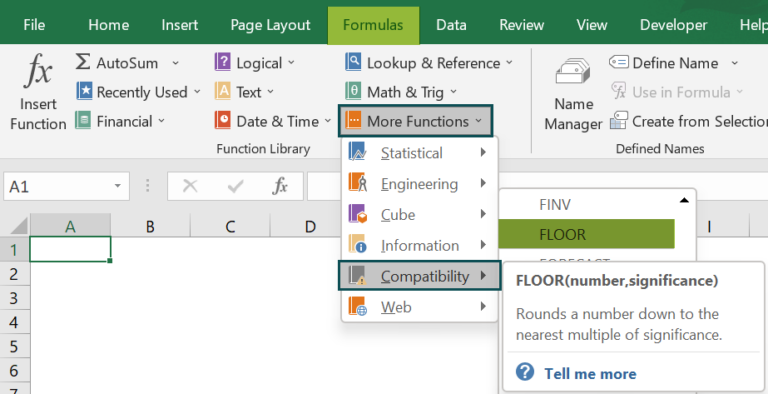# FLOOR Function in ExcelArticle byExcelMojo Team## What is FLOOR Function in Excel?

The FLOOR Function in Excel rounds the specified number to the nearest provided multiple. It is the same as the MROUND function, but the FLOOR function always rounds down. The FLOOR Function accepts two parameters: the number and the significance.

For example, consider the below table with a value in cell A1. We need to use the below steps to obtain the result with FLOOR function.

The steps used to round values with FLOOR function in Excel are as follows:

• Step 1: First, select the cell to display the result. In this example, we have selected cell A1.
• Step 2: Next, enter the FLOOR formula in cell A1.

The complete formula is =FLOOR(11,2).

• Step 3: Then, press Enter key.

We can clearly see that the function has returned the value as 10.

Similarly, we can use FLOOR function to round values.

###### Key Takeaways
• The FLOOR function round down the value towards zero. In simple words, the function returns the lower value to the nearest significant factor.
• The syntax of the FLOOR Excel Function is =FLOOR(number, significance) where both the arguments are mandatory.
• The number argument represents the numeric value that rounds to an exact multiple.
• The significance parameter represents the multiple to the specified number that should be rounded down
• The “#NUM!” error occurs when the number is positive, and the significance is a negative value.

### FLOOR() Excel Formula

The syntax of the FLOOR Excel Function is

• number: This indicates the numeric value that rounds to an exact multiple.
• significance: The significance parameter represents the multiple to the specified number that should be rounded down.

Unlike other functions, both the arguments in the function are mandatory.

### How to Use FLOOR Excel Function?

We can insert FLOOR function in excel using the below two methods.

#### Method #1 – Access from the Excel Ribbon

• Choose the empty cell which will contain the result.
• Go to the Formulas tab.
• Select the More Functions option from the drop-down menu.
• Select the Compatibility option from the menu.
• Select FLOOR from the drop-down menu.
• Meanwhile, a window called Function Arguments appears.
• As the number of arguments, enter the value in the number & significance.
• Finally, select OK.

#### Method #2 – Enter the Worksheet Manually

• Select an empty cell for the output.
• Type =FLOOR( in the selected cell. Alternatively, type =F and double-click the FLOOR function from the list of suggestions shown by Excel.
• Press the Enter key.

The below table shows values and significance in columns A and B. We need to use the below steps to round values using FLOOR function in excel.

The steps to round the value by the FLOOR Function in Excel are as follows:

1. To begin with, select the cell where we will enter the formula and calculate the result. The selected cell, in this case, is cell C2.

2. Next, we will enter the FLOOR formula in cell C2.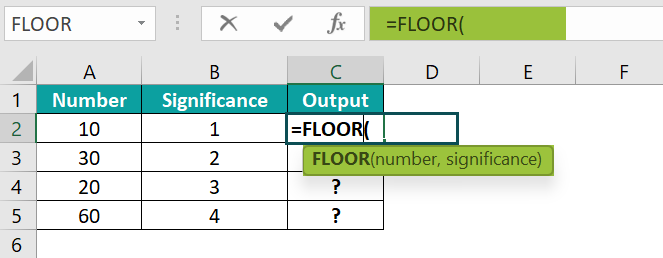3. Then, enter the value of ‘number’ as A2, i.e., the number value.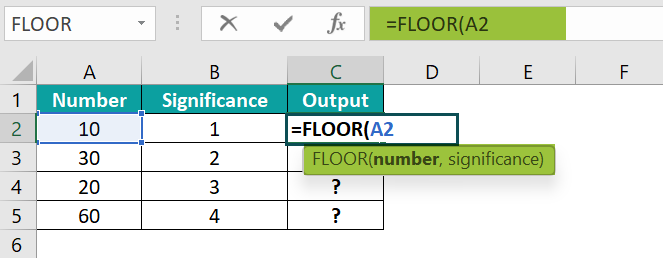4. Also, enter the value of ‘significance’ as B2, i.e., the significance value.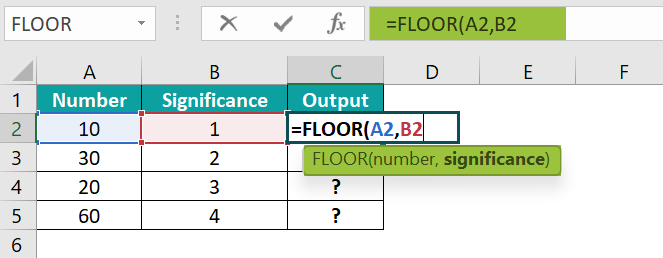5. So, the complete formula is =FLOOR(A2,B2) in cell C2.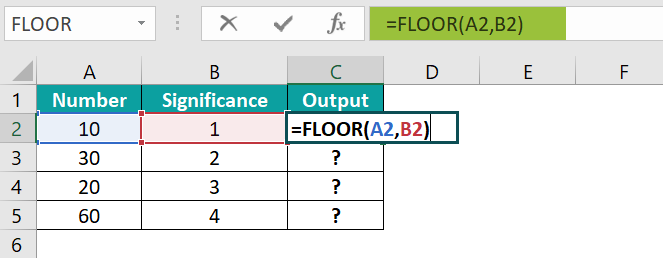6. Press Enter key.

The function has returned the result in cell C2, as shown in the following image.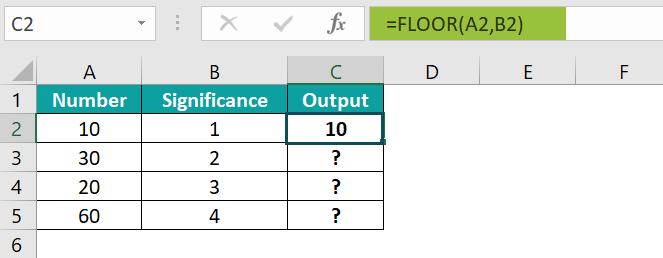7. Drag the cursor to cell C5.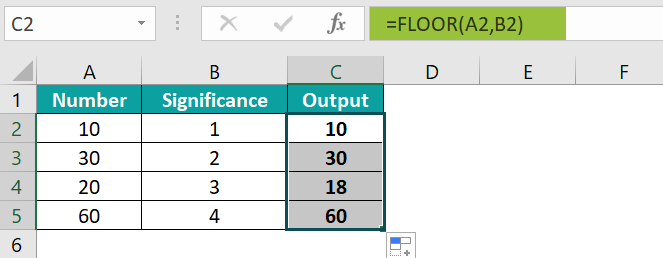Likewise, using FLOOR function in Excel, we can round the values with the nearest provided multiple.

### Examples

#### Example #1

The following table shows products, prices, and their significance in columns A, B, and C, respectively. Now, we need to use the below steps to round the values using FLOOR Function.

The steps to round the value using FLOOR Function in Excel are as follows:

• Step 1: First, select the cell where we will enter the formula and calculate the result. The selected cell, in this case, is cell D2.
• Step 2: Next, we will enter the FLOOR excel formula in cell D2.
• Step 3: Then, enter the value of ‘number’ as B2, i.e., the number value.
• Step 4: Also, enter the value of ‘significance’ as C2, i.e., the significance value.
• Step 5: So, the complete formula is =FLOOR(B2,C2)in cell D2.
• Step 6: Press Enter key.

The function has returned the result in cell D2 as 11.5.

• Step 7: Next, drag the cursor to cell D5, as shown in the following image.

Likewise, we can use FLOOR Function to round the values.

#### Example #2

The below table shows numbers and significance in columns A and B, respectively. Now, we need to use the below steps to calculate the rounded values using Excel REPLACE Function.

The steps to round the value by the FLOOR Function in Excel are as follows:

• Step 1: To begin with, select the cell where we will enter the formula and calculate the result. The selected cell, in this case, is cell C2.
• Step 2: Next, we will enter the FLOOR formula in cell C2.
• Step 3: Then, enter the value of ‘number’ as A2, i.e., the number value.
• Step 4: Now, enter the value of ‘significance’ as B2, i.e., the significance value.
• Step 5: So, the complete formula is =FLOOR(A2,B2)in cell C2.
• Step 6: Press Enter key.

The function has returned the result in cell C2 as 5.2.

• Step 7: Next, drag the cursor to cell C5, as shown in the following image.

Similarly, we can use FLOOR function to obtain the values.

#### Example #3

The following table shows numbers and significance in columns A and B, respectively. Also, we need to use the below steps to calculate rounded values using FLOOR function in Excel.

The steps to round the value by the FLOOR Function in Excel are as follows:

• Step 1: First, select the cell where we will enter the formula and calculate the result. The selected cell, in this case, is cell C2.
• Step 2: Next, we will enter the FLOOR formula in cell C2.
• Step 3: Then, enter the value of ‘number’ as A2, i.e., the number value.
• Step 4: Also, enter the value of ‘significance’ as B2, i.e., the significance value.
• Step 5: So, the complete formula is =FLOOR(A2,B2)in cell C2.
• Step 6: Now, press the Enter key.

The function has returned the result in cell C2, as shown in the below image.

• Step 7: Next, drag the cursor to cell C5, as shown in the following image.

From the obtained results, we know that

• Cell C2 shows #NUM! error that occurs if a negative value is allotted to the significance argument.
• Cell C3 indicates #DIV/0! error that occurs if a zero value is allotted to the significance argument.
• Cell C4 has #VALUE! error if the string value is allotted to the number argument.
• Cell C5 shows -8 since a negative value is allotted to the number argument.

### Important Things to Note

• The ‘number’ & ‘significance’ arguments have different signs. The FLOOR function returns an #VALUE! Error.
• If the number argument equals an exact multiple of significance, no rounding occurs.
• The ‘number’ & ‘significance’ arguments are non-numeric; the FLOOR function returns an #VALUE! Error.
• The ‘number’ argument is a positive value, and the ‘significance’ argument is ‘0’ or ZERO; it returns #DIV/0! Error.
• The previous version of Excel 2003 and 2007, the number and the significant values have the same sign; if not, the Excel FLOOR function would return an error.

1. What does the FLOOR function do in Excel?

The FLOOR function returns a number rounded down based on a considerable significance in Excel. The function is a built-in function in Excel. The function can be entered as part of a formula in a worksheet cell.

A number is rounded either up or down, which is greater than or less than the value. Thus, the rounded-up number has an equal or greater value than the previous number. Similarly, if a number is rounded down, it is comparably lower than the actual number.

The FLOOR Function in Excel always rounds the value down towards zero and returns a numeric value. The FLOOR Function is in the list of the basic rounding functions in Excel.

The syntax of the FLOOR Function in Excel is =FLOOR(number,significance).

2. How to apply the FLOOR function in Excel?

To apply the FLOOR Function in Excel by following the below steps;

First, select an empty cell for the output.
Next, type =FLOOR() in the selected cell. Alternatively, type =F and double-click the FLOOR function from the list of suggestions shown by Excel.
Then, press Enter key.

For example, consider the below table with a value in cell A1. Now, we need to use the below steps to obtain the result using FLOOR function.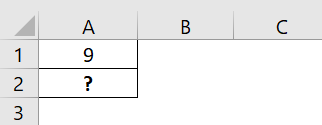The steps used to round values using FLOOR function in Excel are as follows:

Step 1: First, select the cell to display the result. In this example, we have selected cell A2.
Step 2: Next, enter the FLOOR excel formula in cell A2.
So, the complete formula is =FLOOR(11,0).
Step 3: Then, press Enter key.
Clearly, we can see that the function has returned the value as #DIV/0! error because the significance argument value is ‘0’.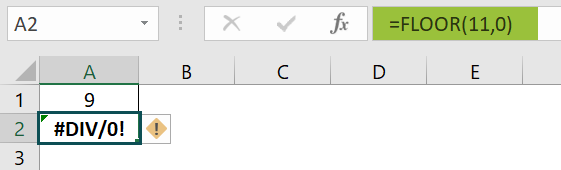Similarly, we can use FLOOR function in Excel to round values.

3. Where is the FLOOR function in Excel?

We can insert FLOOR Function in Excel using the following steps:

To begin with, choose the empty cell which will contain the result.
Next, go to the Formulas tab.
Then, select More Functions option from the drop-down menu.
Now, select Compatibility option from the menu.
Also, select FLOOR from the drop-down menu.
A window called Function Arguments appears.
As the number of arguments, enter the value in the number & significance
Select OK.